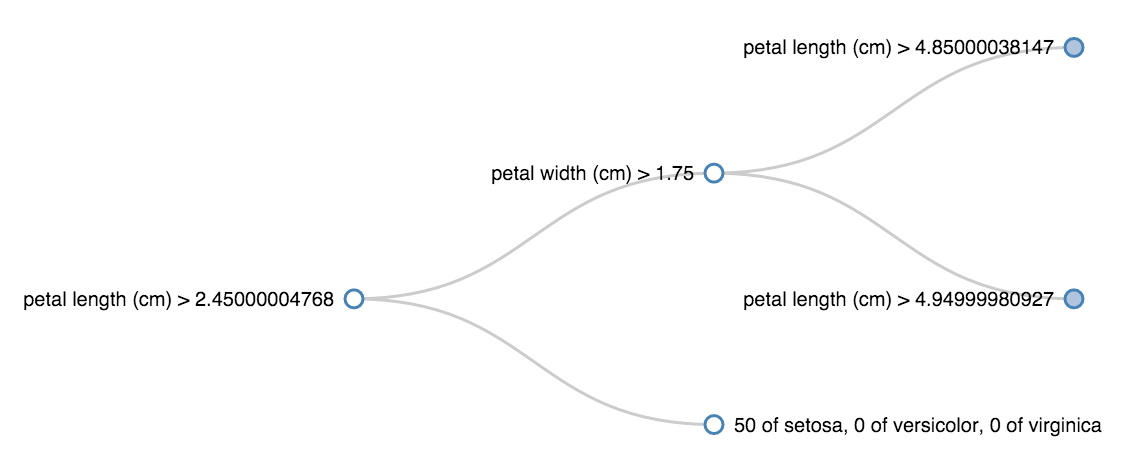# See sklearn trees with D3

Sunday November 29, 2015

The decision trees from scikit-learn are very easy to train and predict with, but it's not easy to see the rules they learn. The code below makes it easier to see inside `sklearn` classification trees, enabling visualizations that look like this:This shows, for example, that all the irises with `petal length (cm)` less than 2.45 were `setosa`.

The ability to interpret the rules of a decision tree is often considered a strength of the algorithm, and in R you can usually `summary()` and `plot()` a tree fit to see the rules. In Python with `sklearn`, there is `export_graphviz`, but it isn't terribly convenient. It shouldn't be so hard to see what's going on inside a tree.

The following JSON format is simple and works with common D3 tree graphing code, so let's target this format:

``````{name: "container thing",
children: [{name: "leaf thing one"},
{name: "leaf thing two"}]}``````

Each `name` will describe a true/false decision rule for an inner node or the distribution of training example labels for a leaf node. The first of a pair of `children` is where the rule is true, and the second is where the rule is false. (These are binary trees.)

The way `sklearn` trees store their rules internally is described in `_tree.pyc`. The `rules` function here examines a fit `sklearn` decision tree to generate a Python dictionary (with structure like the above) representing the decision tree's rules:

``````def rules(clf, features, labels, node_index=0):
"""Structure of rules in a fit decision tree classifier

Parameters
----------
clf : DecisionTreeClassifier
A tree that has already been fit.

features, labels : lists of str
The names of the features and labels, respectively.

"""
node = {}
if clf.tree_.children_left[node_index] == -1:  # indicates leaf
count_labels = zip(clf.tree_.value[node_index, 0], labels)
node['name'] = ', '.join(('{} of {}'.format(int(count), label)
for count, label in count_labels))
else:
feature = features[clf.tree_.feature[node_index]]
threshold = clf.tree_.threshold[node_index]
node['name'] = '{} > {}'.format(feature, threshold)
left_index = clf.tree_.children_left[node_index]
right_index = clf.tree_.children_right[node_index]
node['children'] = [rules(clf, features, labels, right_index),
rules(clf, features, labels, left_index)]
return node``````

How is this used? Let's get a quick example decision tree and take a look:

``````from sklearn.datasets import load_iris
from sklearn.tree import DecisionTreeClassifier

clf = DecisionTreeClassifier(max_depth=3)
clf.fit(data.data, data.target)

rules(clf, data.feature_names, data.target_names)``````

The `rules` function returns the following Python dictionary, formatted for readability here:

``````{'name': 'petal length (cm) > 2.45000004768',
'children': [
{'name': 'petal width (cm) > 1.75',
'children': [
{'name': 'petal length (cm) > 4.85000038147',
'children': [
{'name': '0 of setosa, 0 of versicolor, 43 of virginica'},
{'name': '0 of setosa, 1 of versicolor, 2 of virginica'}]},
{'name': 'petal length (cm) > 4.94999980927',
'children': [
{'name': '0 of setosa, 2 of versicolor, 4 of virginica'},
{'name': '0 of setosa, 47 of versicolor, 1 of virginica'}]}]},
{'name': '50 of setosa, 0 of versicolor, 0 of virginica'}]}``````

This is pretty readable, but now we can also write the result out to a file and visualize it with D3:

``````import json

r = rules(clf, data.feature_names, data.target_names)
with open('rules.json', 'w') as f:
f.write(json.dumps(r))``````

Check out the interactive view! Once again, a partially expanded view looks like this: